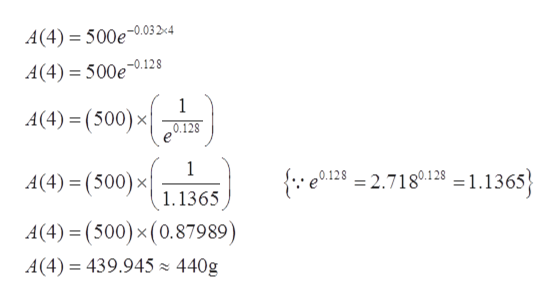# I1the population toA.Evaluatey= 2e )2. When will the population reach 3 million?B. Solve 2e.026.3. How large will the population be in 3 yr?C.Evaluate y= 2e023)4. How large will the population be in 4 months?D.Solve 2e.02 3CONCEPT PREVIEW Radioactive Decay Strontium-90 decays according to the expo-nential functionyyoe 0.0241where t is time in years. Match each question in Column I with the correct procedure inColumn Il to answer thequestion.III5. If the initial amount of Strontium-90 is 200 g,A.Solve 0.75yo yoe0.0241thow much will remain after 10 yr?6. If the initial amount of Strontium-90 is 200 g,how much will remain after 20 yr?B.Evaluate y = 200e .0241 (10)7. What is the half-life of Strontium-90?C. Solve yo yoe 0.0241r.8. How long will it take for any amount ofStrontium-90 to decay to 75% of its initialD.Evaluate y = 200e 0.0241 (20)amount?(Modeling)exponential or logarithmic models. See Examples 1-6.The exercises in this set are grouped according to discipline. They involvePhysical Sciences (Exercises 9-28)An initial amount of a radioactive substance yo is given, along with information aboutthe amount remaining after a given time t in appropriate units. For an equation of theform y = yo ekt that mode ls the situation, give the exact value of k in terms of naturallogarithms.After 6 hr, 10 g remain.10. Уo -30gs9. yo= 60 g; After 3 hr, 20 g remain.12. yo = 20 mg; The half-life is 200 days.11. yo = 10 mg; The half-life is 100 days.14. yo= 8.1 kg; After 4 yr, O.9 kg remains.2.4 lb; After 2 yr, 0.6 lb remains.13. yo15. Decay of Lead A sample of 500 g of radioactive lead-210 decays to polonium-210according to the functionSolve each problem.A(t) = 500e 0.032;where t is time in years. Find the amount of radioactive lead remaining after(c) 20 yr.(d) Find the half-life.(b) 8 yr,(а) 4 yr,ల

Question

15.

How do you answer part (a) and (d)?

check_circle

Step 1

Given that

A sample of 500 g of radioactive lead-210 decay to polonium-210 according the function as

Step 2

To find amount of lead remaining after

a)  4 years

Step 3

Plugging the value t = 4 in in the equation (1)

Then

amount of lea...help_outlineImage TranscriptioncloseA(4) = 500e.03 D:4 A(4) 500e.128 1 A(4)(500) x O.128 1 A(4) (500) x {. 012=2.718123 =1.1365 1.1365 A(4)(500) x (0.87989) A(4) 439.945 440g fullscreen

### Want to see the full answer?

See Solution

#### Want to see this answer and more?

Solutions are written by subject experts who are available 24/7. Questions are typically answered within 1 hour.*

See Solution
*Response times may vary by subject and question.
Tagged in

### Exponents